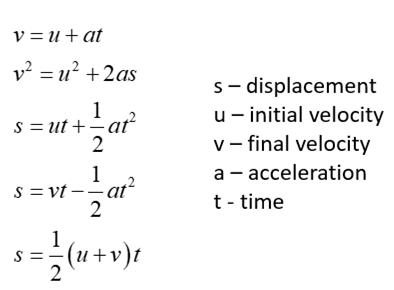## Motion Measurements

Explain the difference between Acceleration, Velocity, and Displacement as measurements.

Hint
The SI unit for displacement is meter ( $$m$$ ).
The SI unit for velocity is meter per second ( $$m/s$$ ).
The SI unit for acceleration is meter per squared second ( $$m/s^2$$ ).
Acceleration – a vector that shows direction and magnitude of changes in velocity Displacement – a vector that shows an initial position of an object to its final position Velocity – a vector that shows direction and rate of motion

The equations of motion describe the relationships between these three concepts extremely well.Acceleration – a vector that shows direction and magnitude of changes in velocity Displacement – a vector that shows an initial position of an object to its final position Velocity – a vector that shows direction and rate of motion